# 1. 2. (1 point) Consider the following convergent series: Suppose that you want to approximate the...

1.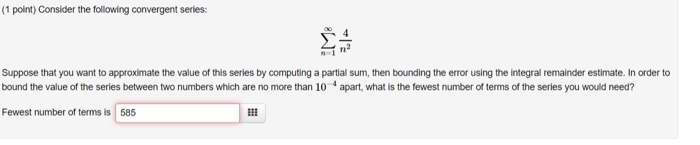2.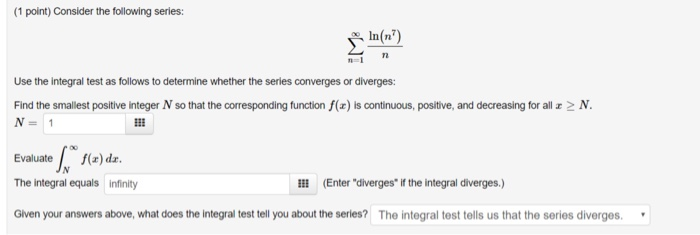(1 point) Consider the following convergent series: Suppose that you want to approximate the value of this series by computing a partial sum, then bounding the error using the integral remainder estimate. In order to bound the value of the series between two numbers which are no more than 10 apart, what is the fewest number of terms of the series you would need? Fewest number of terms is 585
(1 point) Consider the following series: le(n Use the integral test as follows to determine whether the series converges or diverges: Find the smallest positive integer N so that the corresponding function f(x) is continuous, positive, and decreasing for all r > N. N = 1 Evaluate f(a) da. The integral equals Infinity !!! (Enter "diverges if the integral diverges.) Given your answers above, what does the integral test tell you about the series? The integral test tells us that the series diverges.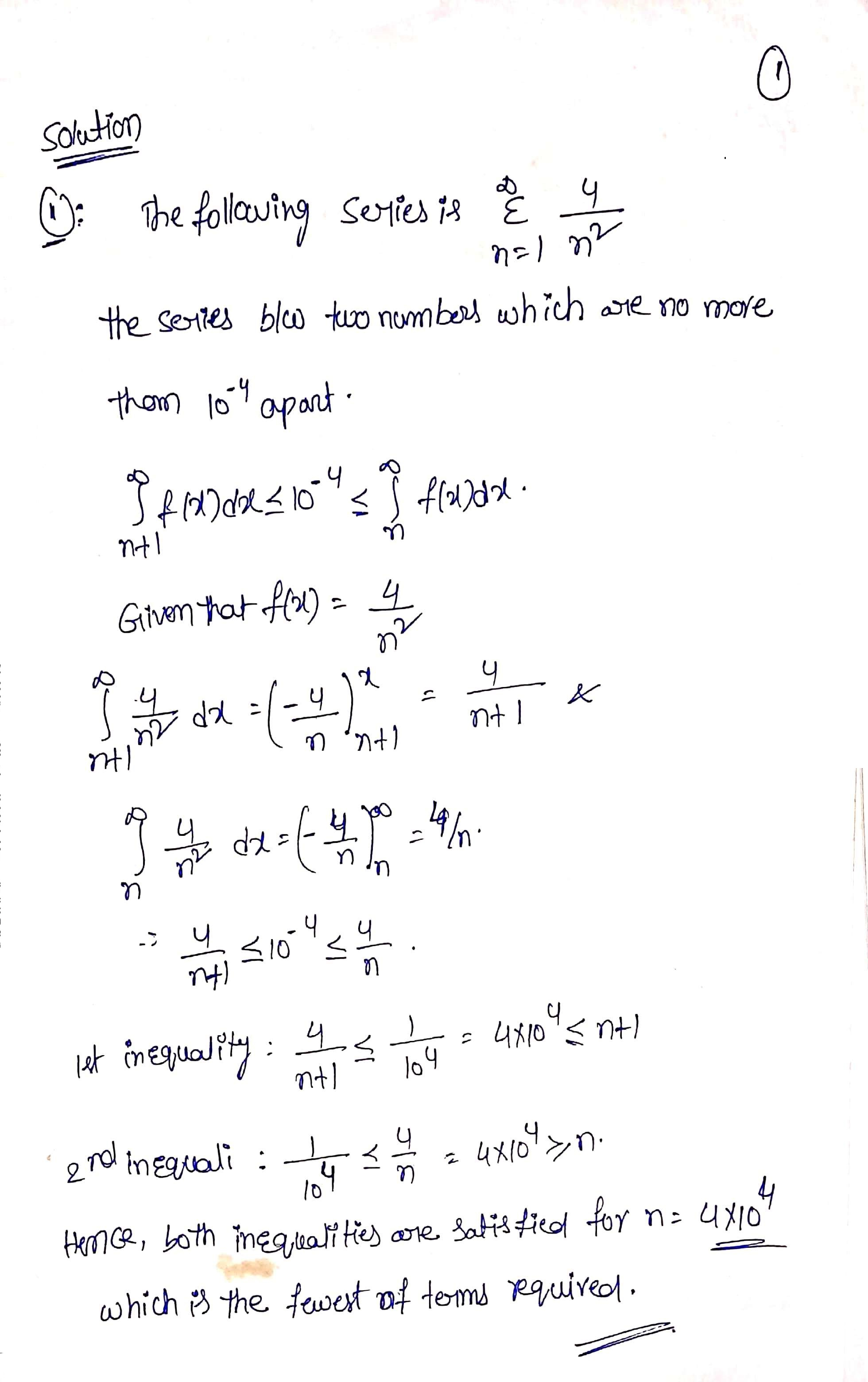#### Earn Coin

Coins can be redeemed for fabulous gifts.

Similar Homework Help Questions
• ### To test the series e 2n for convergence, you can use the Integral Test. (This is...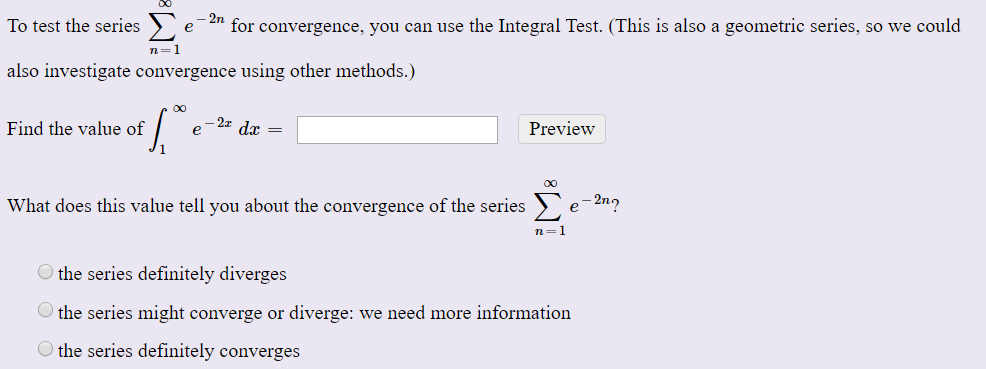To test the series e 2n for convergence, you can use the Integral Test. (This is also a geometric series, so we could n=1 also investigate convergence using other methods.) Find the value of e-24 dx = Preview Ji What does this value tell you about the convergence of the series e-2n? the series definitely diverges the series might converge or diverge: we need more information the series definitely converges Compute the value of the following improper integral, if it...

• ### (1 point) Use the Integral Test to determine whether the infinite series is convergent. 6ne Fill in the correspondi...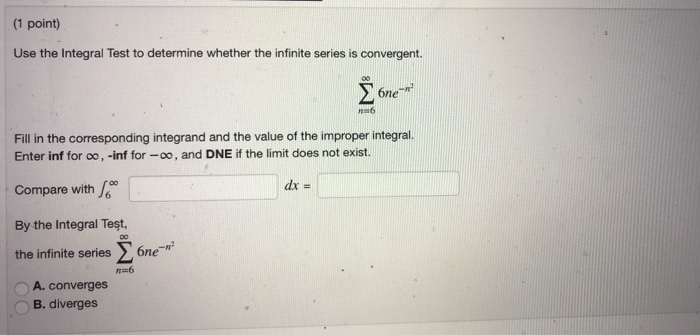(1 point) Use the Integral Test to determine whether the infinite series is convergent. 6ne Fill in the corresponding integrand and the value of the improper integral. Enter inf for oo, -inf for-oo, and DNE if the limit does not exist. Compare with By the Integral Test, the infinite series Σ 6ne" n=6 A. converges - 'B, diverges (1 point) Use the Integral Test to determine whether the infinite series is convergent. 6ne Fill in the corresponding integrand and the...

• ### (1 point) Consider the series an where n=1 an= vn + 6(n+7) en +7 In this...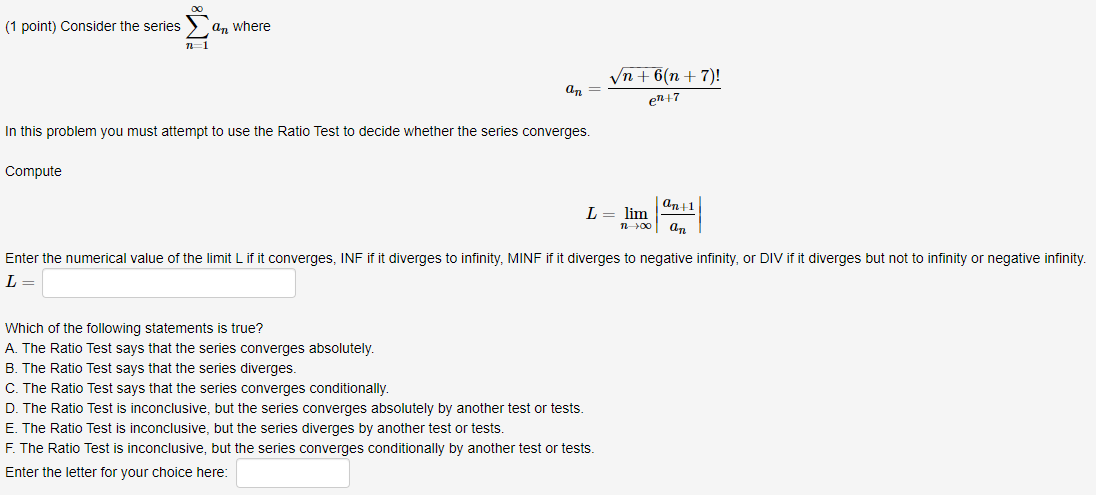(1 point) Consider the series an where n=1 an= vn + 6(n+7) en +7 In this problem you must attempt to use the Ratio Test to decide whether the series converges. Compute L= lim an+1 an n> Enter the numerical value of the limit Lif it converges, INF if it diverges to infinity, MINF if it diverges to negative infinity, or DIV if it diverges but not to infinity or negative infinity. L= Which of the following statements is true?...

• ### (1 point) Consider the series an where n1 an (-1)"n? n2 + 4n + 3 In...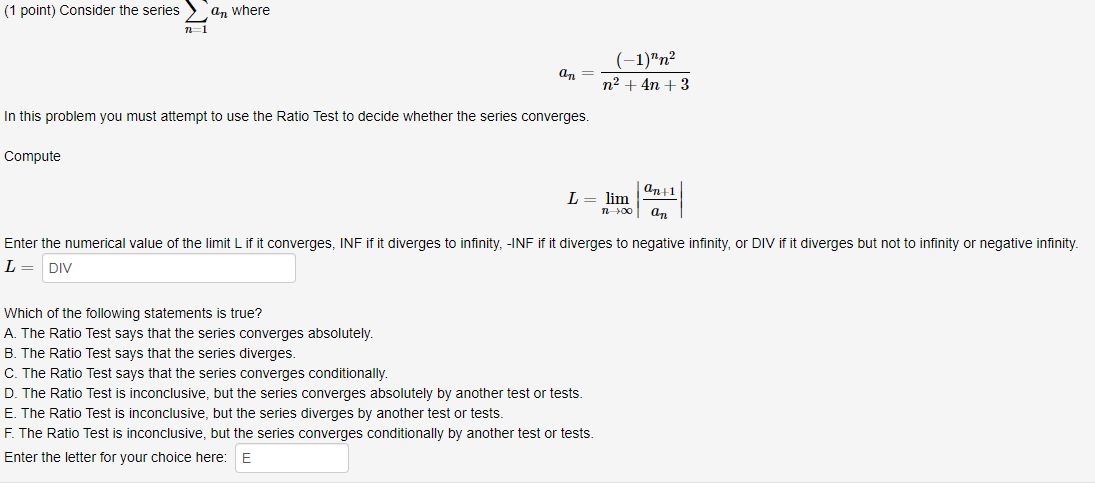(1 point) Consider the series an where n1 an (-1)"n? n2 + 4n + 3 In this problem you must attempt to use the Ratio Test to decide whether the series converges. Compute L= lim an+1 an 1200 Enter the numerical value of the limit Lif it converges, INF if it diverges to infinity, -INF if it diverges to negative infinity, or DIV if it diverges but not to infinity or negative infinity L= DIV Which of the following statements...

• ### IM (1 point) Consider the series an where Vn+5(n+8) a,= e-4 In this problem you must...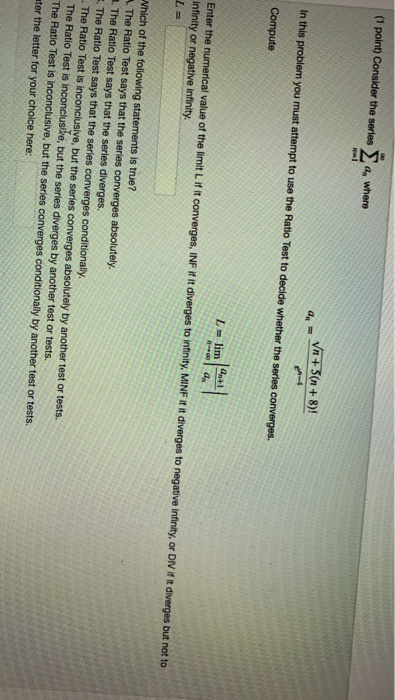IM (1 point) Consider the series an where Vn+5(n+8) a,= e-4 In this problem you must attempt to use the Ratio Test to decide whether the series converges Compute L- lim Enter the numerical value of the limit L if it converges, INF if it diverges to infinity, MINF it it diverges to negative infinity, or DIV if it diverges but not to infinity or negative infinity. Which of the following statements is true? A The Ratio Test says that...

• ### (1 point) Consider the series 5+(-1)"n3 6n3 – In n=1 Which of the following statements accurately...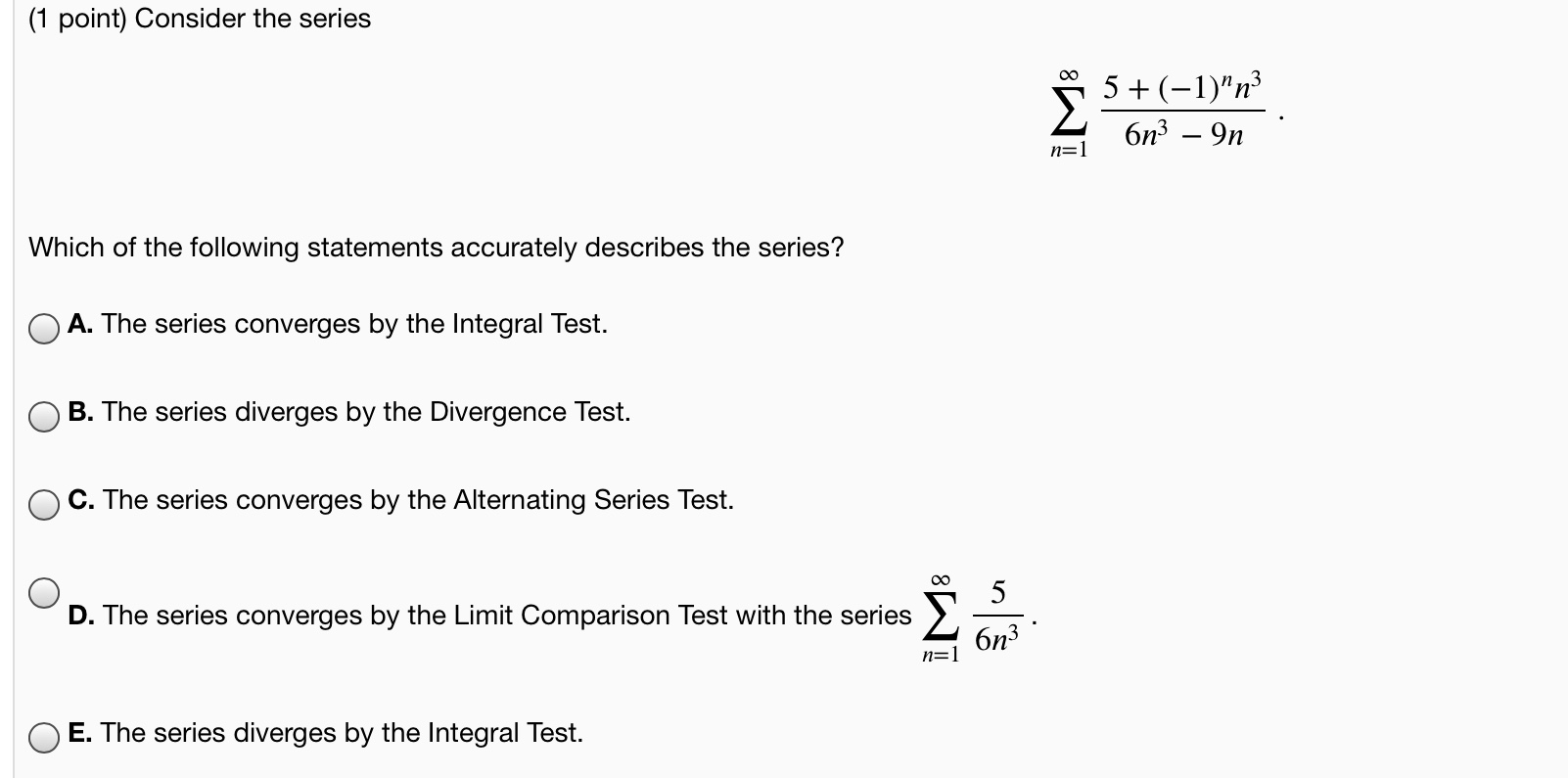(1 point) Consider the series 5+(-1)"n3 6n3 – In n=1 Which of the following statements accurately describes the series? A. The series converges by the Integral Test. B. The series diverges by the Divergence Test. C. The series converges by the Alternating Series Test. 8W 5 D. The series converges by the Limit Comparison Test with the series 6 6n3 n=1 O E. The series diverges by the Integral Test.

• ### (1 point) Consider the series 5+(-1)"n3 6n3 – In n=1 Which of the following statements accurately...(1 point) Consider the series 5+(-1)"n3 6n3 – In n=1 Which of the following statements accurately describes the series? A. The series converges by the Integral Test. B. The series diverges by the Divergence Test. C. The series converges by the Alternating Series Test. 8W 5 D. The series converges by the Limit Comparison Test with the series 6 6n3 n=1 O E. The series diverges by the Integral Test.

• ### (1 point) Consider the senes a, where -1 (-5n+3) a (-n-8) In this problem you must attempt to use the Root Test to...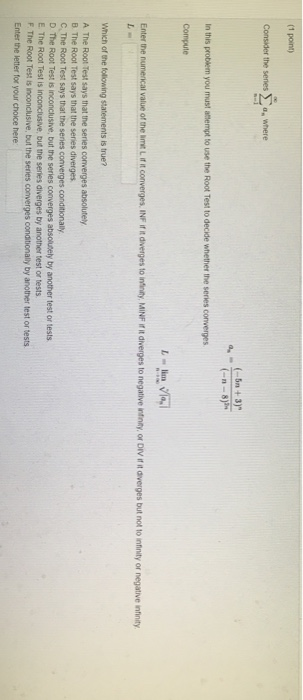(1 point) Consider the senes a, where -1 (-5n+3) a (-n-8) In this problem you must attempt to use the Root Test to decide whether the series converges Compute L-lim Vla Enter the numerncal value of the imit L if it converges, INF if it diverges to infinity, MINF if it diverges to negative ininity, or DIV if it diverges but not to infinity or negative infinity L- Which of the folowing statements is true? A The Root Test says...

• ### (1 point) Consider the series jo 4+(-1)"n? 7n3 - 5 n1 Which of the following statements...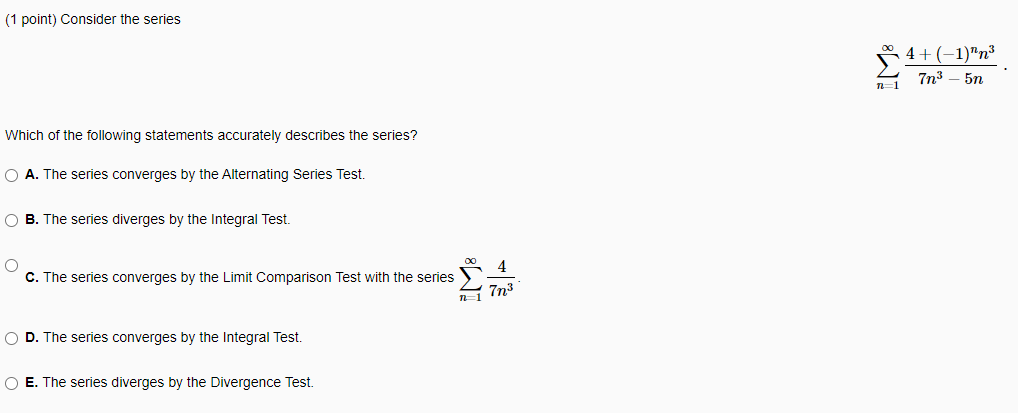(1 point) Consider the series jo 4+(-1)"n? 7n3 - 5 n1 Which of the following statements accurately describes the series? O A. The series converges by the Alternating Series Test. B. The series diverges by the Integral Test. O 4 C. The series converges by the Limit Comparison Test with the series ni 7n3 O D. The series converges by the Integral Test. O E. The series diverges by the Divergence Test.

• ### HW10: Problem 8 Prev Up Next (1 pt) Consider the series Em_1 and where n+4 an...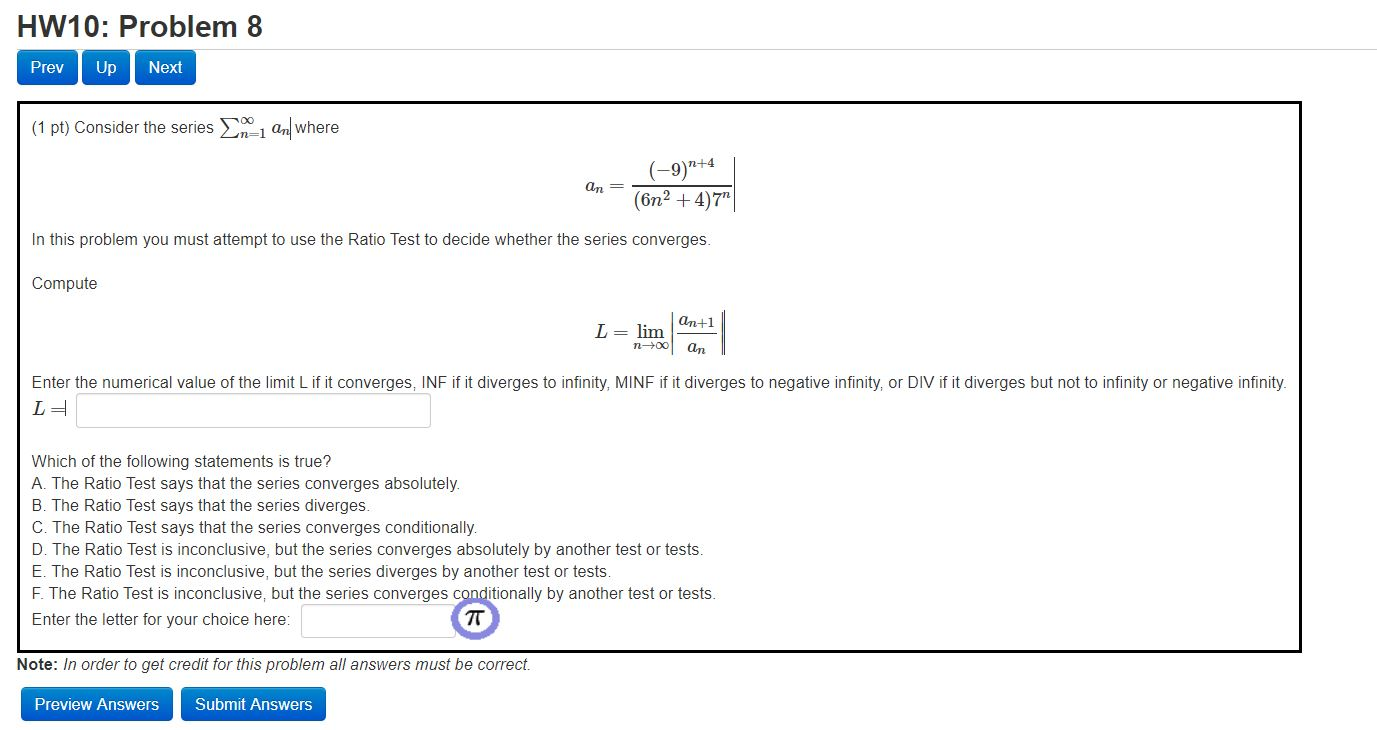HW10: Problem 8 Prev Up Next (1 pt) Consider the series Em_1 and where n+4 an = (-9)" (6n2+4)7" In this problem you must attempt to use the Ratio Test to decide whether the series converges. Compute an+1 L= lim 1-00 an Enter the numerical value of the limit Lif it converges, INF if it diverges to infinity, MINF if it diverges to negative infinity, or DIV if it diverges but not to infinity or negative infinity LE Which of...